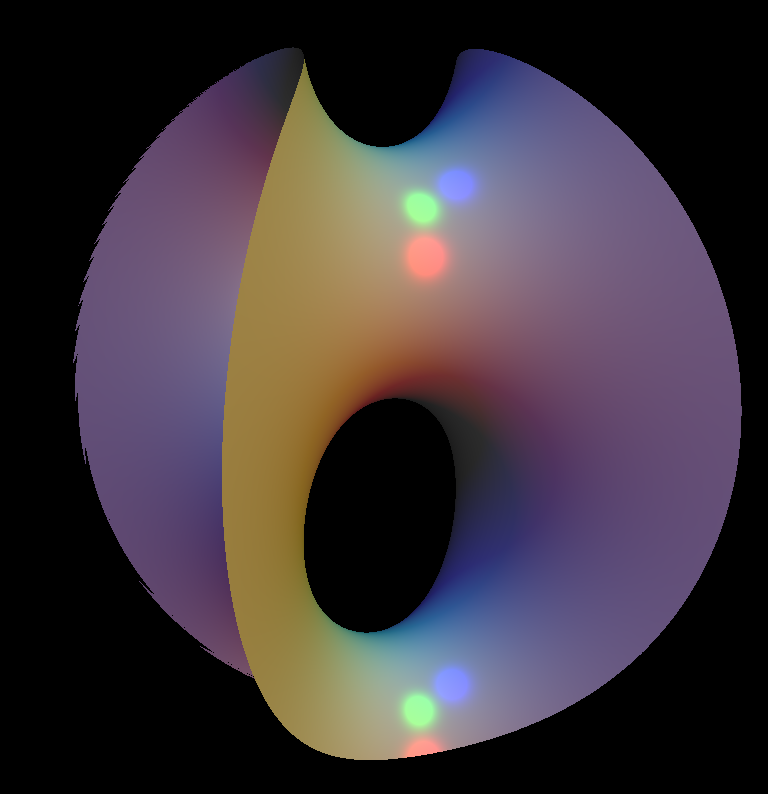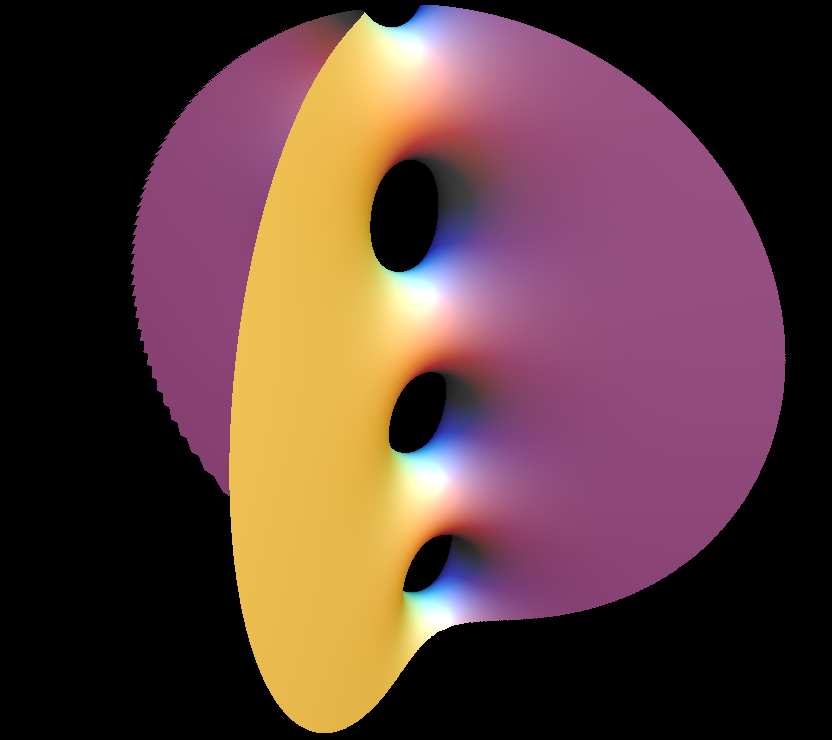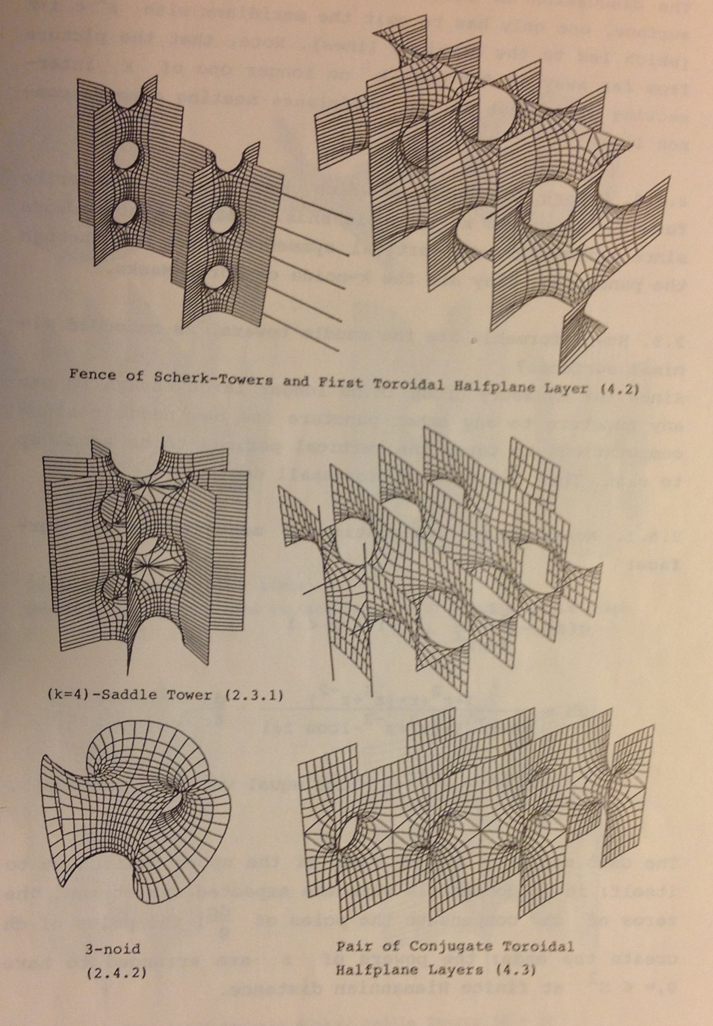# Scherk Surface

It was a sensation when Heinrich Scherk discovered in 1834 new minimal surfaces since during the previous 50 years only the Catenoid and the Helicoid where known. (The plane is a minimal surface, but not counted as interesting.)

Two of his discoveries were without singularities, namely a doubly periodic one which Scherk described as the graph of a function and a singly periodic one which Scherk presented by an implicit equation. (doubly periodic = translational symmetries in two directions, singly periodic = translational symmetries in one direction)

These two surfaces turned out to be fundamental examples when in the 1980s a wealth of unexpected minimal surfaces were found. These modern surfaces are all made with the Weierstrass representation - which leaves Scherk's discoveries as a singular success, since no methods were found to write down functions whose graphs are minimal surfaces nor functions which have one minimal level.

## Scherk's Singly PeriodicScherk's singly periodic minimal surface is conjugate to Scherk's doubly periodic surface. This image shows the portion in a sphere of radius 4.5. It can be described by the implicit equation sin(z) - sinh(x)*sinh(y) = 0 see Saddle Tower for generalizations and renderings made with the Weierstrass representation. We are not aware of images from the 19th century. Our rendering raytraces the solution of the implicit equation.A raytraced image of Scherk's singly periodic surface in a ball of radius 10.

Scherk Minimal Surface Parametric Equations

```  x = u
y = v
z = (ln(cos(aa * v) / cos(aa * u))) / aa```Illustration from 1988. 〔Karcher, H.: Embedded Minimal Surfaces Drived From Scherk's Examples. Manuscripta Math. 62, 83 - 114 (1988)〕Illustration from 1988. 〔Karcher, H.: Embedded Minimal Surfaces Drived From Scherk's Examples. Manuscripta Math. 62, 83 - 114 (1988)〕

#### Related Surface

Get red/blue stereo glasses from amazon

CatalanHennebergScherk.pdf

Get red/blue stereo glasses from amazon